## Legendre Differential Equation

The second-order Ordinary Differential Equation(1)

which can be rewritten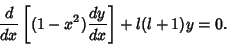(2)

The above form is a special case of the associated Legendre differential equation with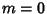. The Legendre differential equation has Regular Singular Points at, 1, and. It can be solved using a series expansion,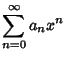(3)(4)(5)

Plugging in,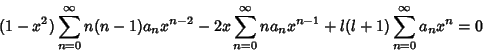(6)(7)(8)(9)(10)

so each term must vanish and(11)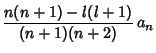(12)

Therefore,(13)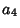(14)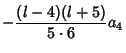(15)

so the Even solution is(16)

Similarly, the Odd solution is(17)

If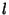is an Even Integer, the seriesreduces to a Polynomial of degreewith only Even Powers ofand the seriesdiverges. Ifis an Odd Integer, the seriesreduces to a Polynomial of degreewith only Odd Powers ofand the seriesdiverges. The general solution for an Integeris given by the Legendre Polynomials(18)

where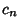is chosen so that. If the variableis replaced by, then the Legendre differential equation becomes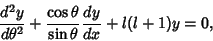(19)

as is derived for the associated Legendre differential equation with.

The associated Legendre differential equation is(20)(21)

The solutions to this equation are called the associated Legendre polynomials. Writing, first establish the identities(22)(23)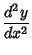(24)

and(25)

Therefore,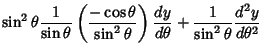(26)

Plugging (22) into (26) and the result back into (21) gives(27)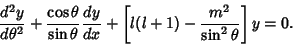(28)

References

Abramowitz, M. and Stegun, C. A. (Eds.). Handbook of Mathematical Functions with Formulas, Graphs, and Mathematical Tables, 9th printing. New York: Dover, p. 332, 1972.